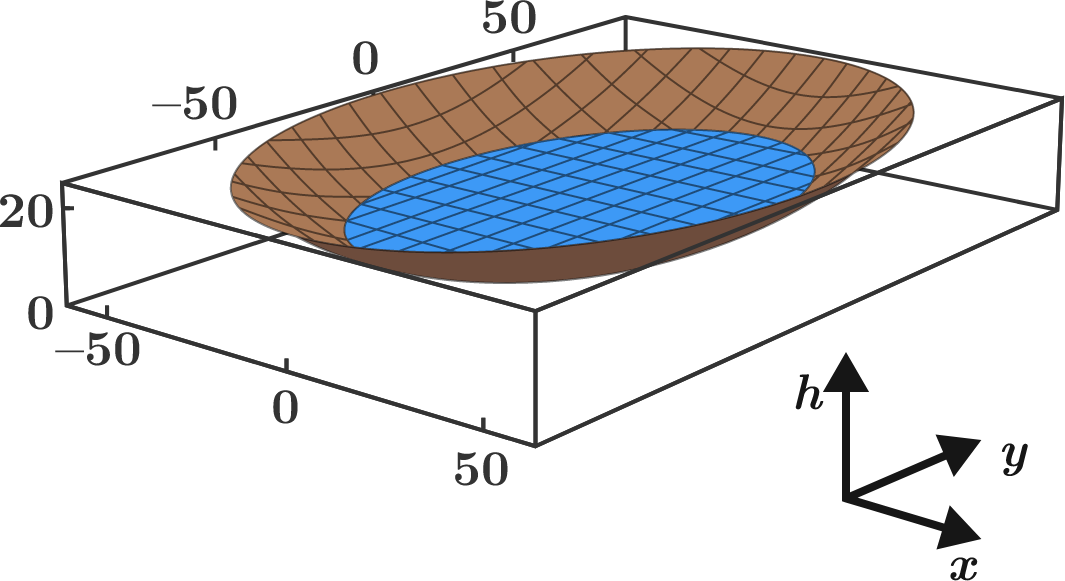# Lake Volume

Calculus Level 3

The height profile of a valley basin can be described by the two-dimensional parabolic function$h(x, y) = \frac{x^2}{144\,\text{m}} + \frac{y^2}{324\,\text{m}}.$ Now the basin is filled by a rainstorm to a height of $h_0 = 12\,\text{m}.$ What is the volume of the resulting lake $($in $\text{m}^3)$ to the nearest integer?Hint: Find the shape of the cross-sectional areas enclosed by the equipotential lines $h(x, y) = z = \text{constant}$. The volume then results from the integral $\displaystyle V = \int_0^{h_0} A (z)\, dz$ over the cross-sectional area $A(z).$

×

Problem Loading...

Note Loading...

Set Loading...